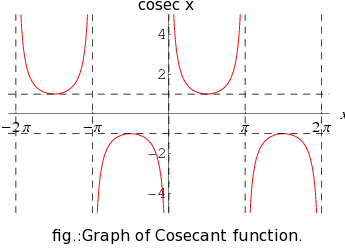Q

# Help me answer: - Trigonometry - JEE Main-5

What is the solution set of the equation   in the interval [ ] ?

• Option 1)

• Option 2)

• Option 3)

• Option 4)

86 Views

As we have learned

Graph of Trigonometric Ratios -- wherein

This is the graph of

As seen in the graph the graph is always on or above the line x = 1 or on below the linbe x =-1

Thus  cosec x > 1 will be  because at x  ;

cosec x = 1

Option 1)

Option 2)

Option 3)

Option 4)

Exams
Articles
Questions